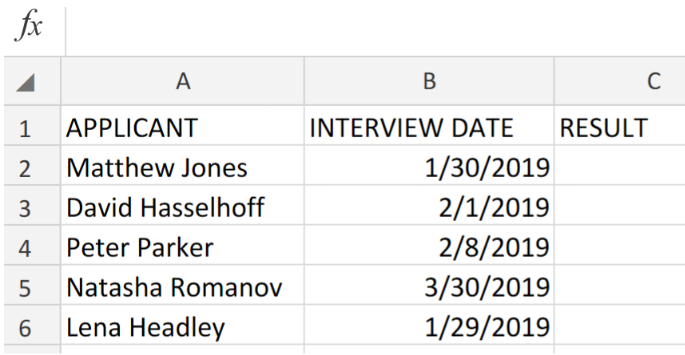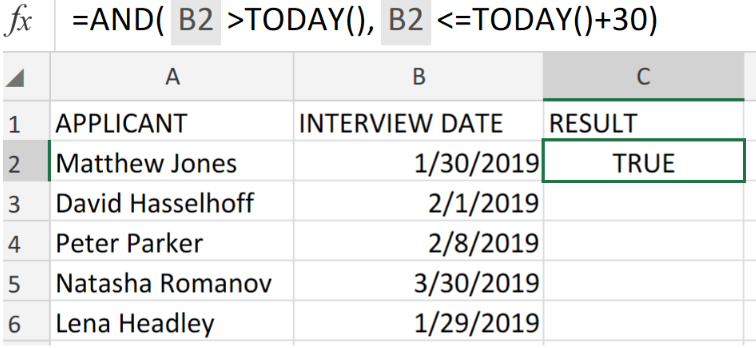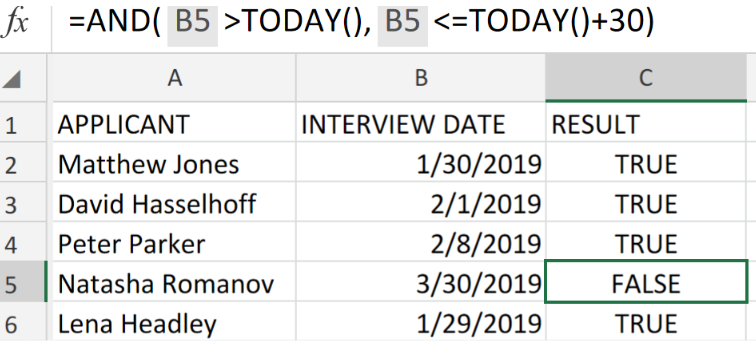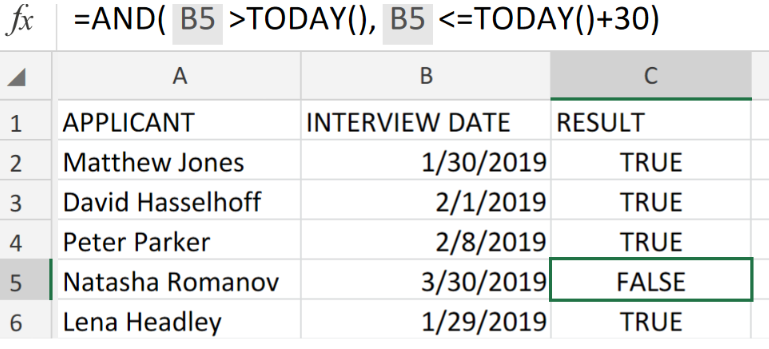Get instant live expert help with Excel or Google Sheets“My Excelchat expert helped me in less than 20 minutes, saving me what would have been 5 hours of work!”

#### Post your problem and you’ll get Expert help in seconds.

Your message must be at least 40 characters
Our professional Expert are available now. Your privacy is guaranteed.

# Excel DATA VALIDATION Using the TODAY and AND FunctionsRead time: 25 minutesFigure 1. Excel DATA VALIDATION Using the TODAY and AND Functions.

Let’s assume that we have to generate a DATA VALIDATION using Excel’s TODAY and AND Functions to permit entries of dates occurring within the next 30 days, we must utilize a custom formula syntax based on the AND and TODAY Functions in Excel.

## Formula Syntax

`=AND(logical1,[logical2], …)`

This formula syntax returns as a result TRUE or FALSE. Which simply means that formula will return FALSE if all conditions in the formula are FALSE, and TRUE when all conditions in the formula are TRUE.

## How to use Excel DATA VALIDATION Using the TODAY and AND Functions.

Let’s say we have to create a data entry validation for dates exceeding 30 days from today in Excel,, we are going to get this done by following 3 simple steps;

1. In our worksheet example below, we have a collection of dates scheduled for interviews;Figure 2. Excel DATA VALIDATION Using the TODAY and AND Functions.

Our purpose here is to flag any date entries that occur after a period of 30 days from the present date.

1. The DATA VALIDATION formula that we will enter into cell C2 of our worksheet example is as follows;

`=AND(B2>TODAY(),B2<=TODAY()+30)`Figure 3. Excel DATA VALIDATION Using the TODAY and AND Functions.

Excel returned the result of the Data Validation as TRUE because the date is within the 30 day period (assume that today is 27th of January 2019)

1. Copy and paste the modified version of the Data Validation formula in cell C2 above, down into the other cells in the RESULT column for the next results.Figure 4. Excel DATA VALIDATION Using the TODAY and AND Functions.

We can use the Excel AND function when we want more than one or both conditions to be met.Figure 5. Final Result.

## Instant Connection to an Expert through our Excelchat Service:

Our live Excelchat Service is here for you. We have Excel Experts available 24/7 to answer any Excel questions you may have. Guaranteed connection within 30 seconds and a customized solution for you within 20 minutes.

### Did this post not answer your question? Get a solution from connecting with the expert.Another blog reader asked this question today on Excelchat:
Related blogs
Solution examplesYes hello Im looking for help on conditional formatting I currently have a conditional format for D9>D32 and so on from D9:S20. I need to add an if statement that references cell D4 if the first four characters are 1358 for the rule to apply can you please assist?
Solved by Z. U. in 20 minscan you teach me the steps to create a nested if function, and to nest an AND function inside of an IF function?
Solved by A. Q. in 22 minsUse the Vlookup Function to complete the "employee" column of table 2. Use "job Id" from table 2 as your lookup_value(s) and table 1 as your reference.
Solved by C. H. in 16 minsI have a list or people who are ranked in numbers from 3 to 6 I need to recognize the contents of each multiple cells and create an equivalent letter. for that value into another column. e.g. Cell E1 = 3 to show in new cell that row (H1) the letter "C" I have multiple rows with different values in column "E" Can this be done?
Solved by I. J. in 30 minsIf a cell in another sheet is populated I need a vlookup done. If the cell is not populated I need the cell to return blank.
Solved by T. D. in 60 mins## Subscribe to Excelchat.coAnother blog reader asked this question today on Excelchat: